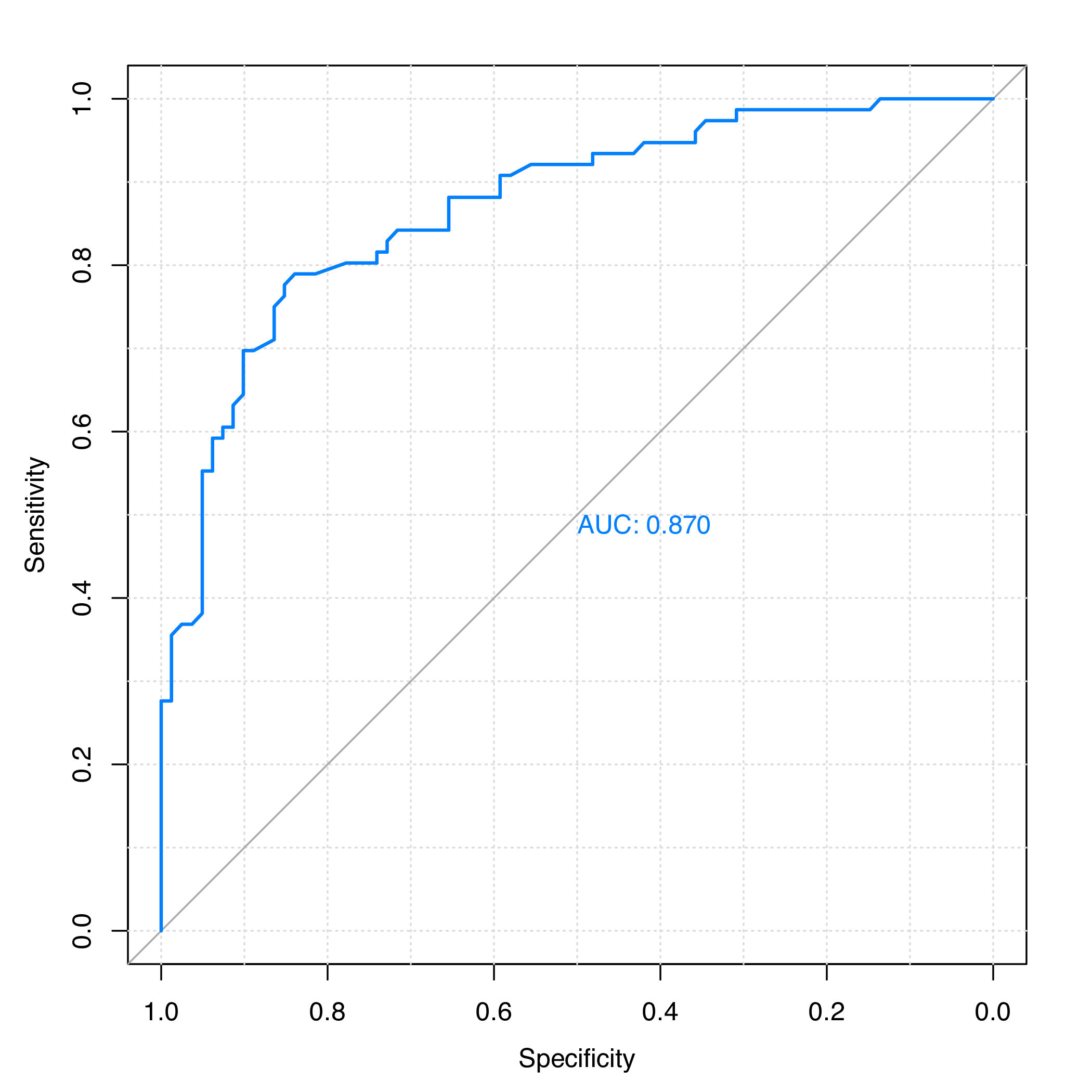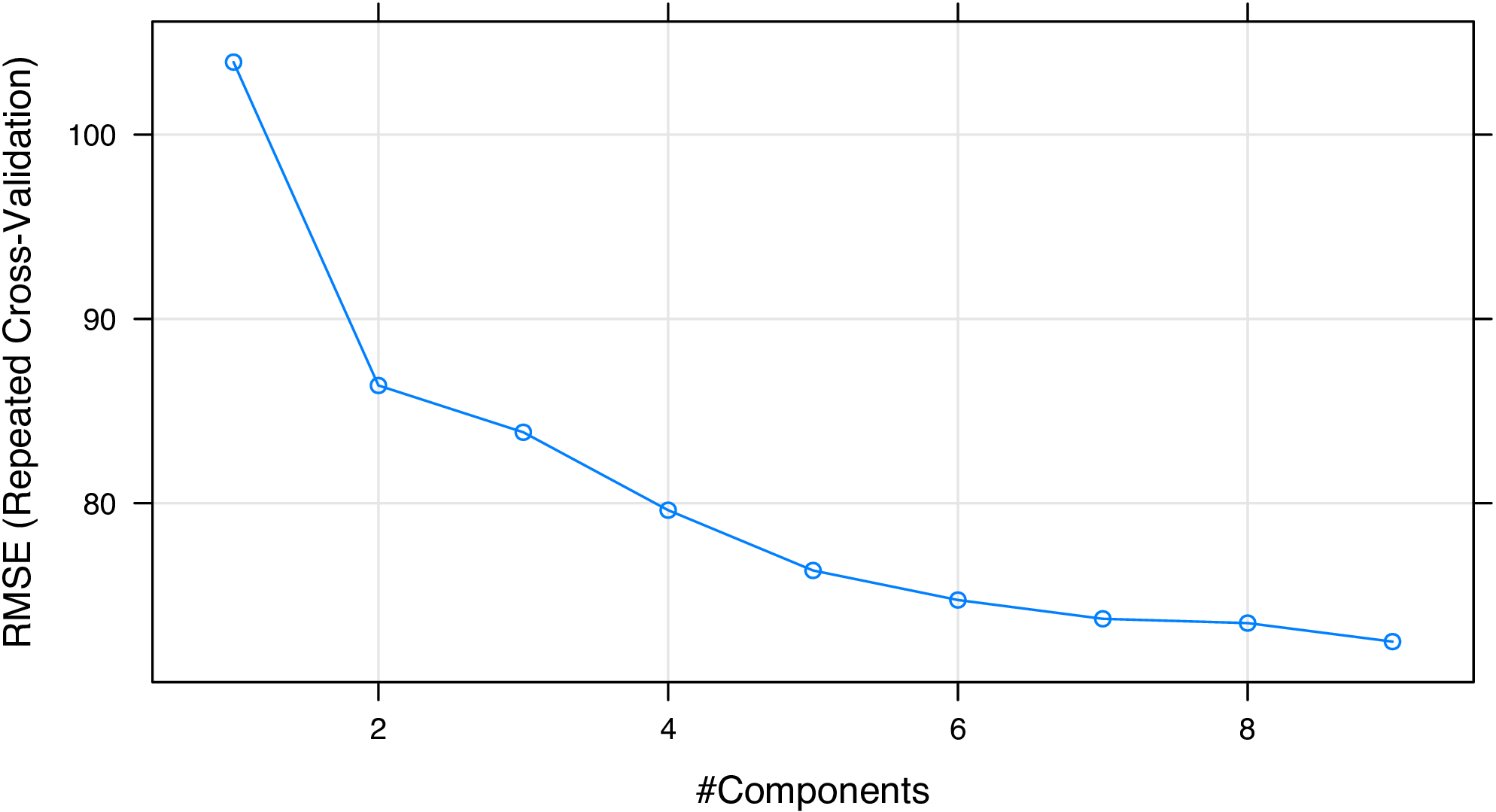# 1 Introduction

The Rcpi package Cao et al. (2015) offers an R/Bioconductor package emphasizing the comprehensive integration of bioinformatics and chemoinformatics into a molecular informatics platform for drug discovery.

Rcpi implemented and integrated the state-of-the-art protein sequence descriptors and molecular descriptors/fingerprints with R. For protein sequences, the Rcpi package can

• Calculate six protein descriptor groups composed of fourteen types of commonly used structural and physicochemical descriptors that include 9,920 descriptors.
• Calculate profile-based protein representation derived by PSSM (Position-Specific Scoring Matrix).
• Calculate six types of generalized scales-based descriptors derived by various dimensionality reduction methods for proteochemometric (PCM) modeling.
• Parallellized pairwise similarity computation derived by protein sequence alignment and Gene Ontology (GO) semantic similarity measures within a list of proteins.

For small molecules, the Rcpi package can

• Calculate 307 molecular descriptors (2D/3D), including constitutional, topological, geometrical, and electronic descriptors, etc.
• Calculate more than ten types of molecular fingerprints, including FP4 keys, E-state fingerprints, MACCS keys, etc., and parallelized chemical similarity search.
• Parallelized pairwise similarity computation derived by fingerprints and maximum common substructure search within a list of small molecules.

By combining various types of descriptors for drugs and proteins in different methods, interaction descriptors representing protein-protein or compound-protein interactions can be conveniently generated with Rcpi, including:

• Two types of compound-protein interaction (CPI) descriptors
• Three types of protein-protein interaction (PPI) descriptors

Several useful auxiliary utilities are also shipped with Rcpi:

• Parallelized molecule and protein sequence retrieval from several online databases, like PubChem, ChEMBL, KEGG, DrugBank, UniProt, RCSB PDB, etc.
• Loading molecules stored in SMILES/SDF files and loading protein sequences from FASTA/PDB files
• Molecular file format conversion

The computed protein sequence descriptors, molecular descriptors/fingerprints, interaction descriptors and pairwise similarities are widely used in various research fields relevant to drug disvery, such as, bioinformatics, chemoinformatics, proteochemometrics and chemogenomics.

## 1.1 Installation

To install the Rcpi package, use:

``````install.packages("BiocManager")
BiocManager::install("Rcpi")``````

To make the Rcpi package fully functional (especially the Open Babel related functionalities), we recommend the users also install the Enhances packages by:

``BiocManager::install("Rcpi", dependencies = c("Imports", "Enhances"))``

Several dependencies of the Rcpi package may require some system-level libraries, check the corresponding manuals of these packages for detailed installation guides.

## 1.2 How to cite Rcpi

If you feel Rcpi is useful in your research, please cite our paper:

Dong-Sheng Cao, Nan Xiao, Qing-Song Xu, and Alex F. Chen. (2015). Rcpi: R/Bioconductor package to generate various descriptors of proteins, compounds and their interactions. Bioinformatics 31 (2), 279-281.

BibTeX entry:

``````@article{Rcpi2015,
author = {Cao, Dong-Sheng and Xiao, Nan and Xu, Qing-Song and Alex F. Chen.},
title = {{Rcpi: R/Bioconductor package to generate various descriptors
of proteins, compounds and their interactions}},
journal = {Bioinformatics},
year = {2015},
volume = {31},
number = {2},
pages = {279--281},
doi = {10.1093/bioinformatics/btu624},
issn = {1367-4803},
url = {http://bioinformatics.oxfordjournals.org/content/31/2/279}
}``````

# 2 Applications in Bioinformatics

For bioinformatics research, Rcpi calculates commonly used descriptors and proteochemometric (PCM) modeling descriptors for protein sequences. Rcpi also computes pairwise similarities derived by GO semantic similarity and sequence alignment.

## 2.1 Predicting protein subcellular localization

Protein subcellular localization prediction involves the computational prediction of where a protein resides in a cell. It is an important component of bioinformatics-based prediction of protein function and genome annotation, and can also aid us to identify novel drug targets.

Here we use the subcellular localization dataset of human proteins presented in the study of Chou and Shen (2008) for a demonstration. The complete dataset includes 3,134 protein sequences (2,750 different proteins), classified into 14 human subcellular locations. We selected two classes of proteins as our benchmark dataset. Class 1 contains 325 extracell proteins, and class 2 includes 307 mitochondrion proteins.

First, we load the Rcpi package, then read the protein sequences stored in two separated FASTA files with `readFASTA()`:

``````library("Rcpi")

# load FASTA files
"vignettedata/extracell.fasta",
package = "Rcpi"
))
"vignettedata/mitochondrion.fasta",
package = "Rcpi"
))``````

The loaded sequences are stored as two lists in R, and each component in the list is a character string representing one protein sequence. In this case, there are 325 extracell protein sequences and 306 mitonchon protein sequences:

``length(extracell)``
``##  325``
``length(mitonchon)``
``##  306``

To assure that the protein sequences only have the twenty standard amino acid types which is required for the descriptor computation, we use the `checkProt()` function to do the amino acid type sanity checking and remove the non-standard protein sequences:

``````extracell <- extracell[(sapply(extracell, checkProt))]
mitonchon <- mitonchon[(sapply(mitonchon, checkProt))]``````
``length(extracell)``
``##  323``
``length(mitonchon)``
``##  304``

Two protein sequences were removed from each class. For the remaining sequences, we calculate the amphiphilic pseudo amino acid composition (APAAC) descriptor Chou (2005) and create class labels for the random forest classification modeling.

``````# calculate APAAC descriptors
x1 <- t(sapply(extracell, extractProtAPAAC))
x2 <- t(sapply(mitonchon, extractProtAPAAC))
x <- rbind(x1, x2)

# make class labels
labels <- as.factor(c(rep(0, length(extracell)), rep(1, length(mitonchon))))``````

In Rcpi, the functions of commonly used descriptors for protein sequences and proteochemometric (PCM) modeling descriptors are named after `extractProt...()` and `extractPCM...()`.

Next, we will split the data into a 75% training set and a 25% test set.

``````set.seed(1001)

# split training and test set
tr.idx <- c(
sample(1:nrow(x1), round(nrow(x1) * 0.75)),
sample(nrow(x1) + 1:nrow(x2), round(nrow(x2) * 0.75))
)
te.idx <- setdiff(1:nrow(x), tr.idx)

x.tr <- x[tr.idx, ]
x.te <- x[te.idx, ]
y.tr <- labels[tr.idx]
y.te <- labels[te.idx]``````

We will train a random forest classification model on the training set with 5-fold cross-validation, using the package.

``````library("randomForest")
rf.fit <- randomForest(x.tr, y.tr, cv.fold = 5)
print(rf.fit)``````

The training result is:

``````## Call:
##  randomForest(x = x.tr, y = y.tr, cv.fold = 5)
##                Type of random forest: classification
##                      Number of trees: 500
## No. of variables tried at each split: 8
##
##         OOB estimate of  error rate: 25.11%
## Confusion matrix:
##     0   1 class.error
## 0 196  46   0.1900826
## 1  72 156   0.3157895``````

With the model trained on the training set, we predict on the test set and plot the ROC curve with the package, as is shown in Figure 1.

``````# predict on test set
rf.pred <- predict(rf.fit, newdata = x.te, type = "prob")[, 1]

# plot ROC curve
library("pROC")
plot.roc(y.te, rf.pred, grid = TRUE, print.auc = TRUE)``````Figure 1: ROC curve for the test set of protein subcellular localization data

The area under the ROC curve (AUC) is:

``````## Call:
## plot.roc.default(x = y.te, predictor = rf.pred, col = "#0080ff",
##                  grid = TRUE, print.auc = TRUE)
##
## Data: rf.pred in 81 controls (y.te 0) > 76 cases (y.te 1).
## Area under the curve: 0.8697``````

# 3 Applications in Chemoinformatics

For chemoinformatics research, Rcpi calculates various types of molecular descriptors/fingerprints, and computes pairwise similarities derived by fingerprints and maximum common substructure search. Rcpi also provides the `searchDrug()` function for parallelized molecular similarity search based on these similarity types.

## 3.1 Regression modeling in QSRR study of retention indices

In Yan et al. (2012), a quantitative structure-retention relationship study was performed for 656 flavor compounds on four stationary phases of different polarities, using constitutional, topological and geometrical molecular descriptors. The gas chromatographic retention indices (RIs) of these compounds were accurately predicted using linear models. Here we choose the molecules and their RIs of one stationary phase (OV101) as our benchmark dataset.

Since it would be rather tedious to implement the complete cross-validation procedures, the R package `caret` is used here. To run the R code below, users need to install the package and the required predictive modeling packages first. The package provides a unified interface for the modeling tuning task across different statistical machine learning packages. It is particularly helpful in QSAR modeling, for it contains tools for data splitting, pre-processing, feature selection, model tuning and other functionalities.

Just like the last section, we load the Rcpi package, and read the molecules stored in a SMILES file:

``````library("Rcpi")

RI.smi <- system.file(
"vignettedata/RI.smi",
package = "Rcpi"
)
RI.csv <- system.file(
"vignettedata/RI.csv",
package = "Rcpi"
)

x.mol <- readMolFromSmi(RI.smi, type = "mol")
x.tab <- read.table(RI.csv, sep = "\t", header = TRUE)
y <- x.tab\$RI``````

The `readMolFromSmi()` function is used for reading molecules from SMILES files, for molecules stored in SDF files, use `readMolFromSDF()` instead.

The CSV file `RI.csv` contains tabular data for the retention indices, compound name, and odor information of the compounds. Here we only extracted the RI values by calling `x.tab\$RI`.

After the molecules were properly loaded, we calculate several selected molecular descriptors. The corresponding functions for molecular descriptor calculation are all named after `extractDrug...()` in `Rcpi`:

``````# calculate selected molecular descriptors
x <- suppressWarnings(cbind(
extractDrugALOGP(x.mol),
extractDrugApol(x.mol),
extractDrugECI(x.mol),
extractDrugTPSA(x.mol),
extractDrugWeight(x.mol),
extractDrugWienerNumbers(x.mol),
extractDrugZagrebIndex(x.mol)
))``````

After the descriptors were calculated, the result `x` will be a data frame, each row represents one molecule, and each column is one descriptor (predictor). The Rcpi package integrated the molecular descriptors and chemical fingerprints calculated by the `rcdk` package Steinbeck et al. (2003) and the ChemmineOB package Horan and Girke (2013).

Next, a partial least squares model will be fitted with the `pls` and the `caret` package. The cross-validation setting is 5-fold repeated cross-validation (repeat for 10 times).

``````# regression on training set
library("caret")
library("pls")

# cross-validation settings
ctrl <- trainControl(
method = "repeatedcv", number = 5, repeats = 10,
summaryFunction = defaultSummary
)

# train a pls model
set.seed(1002)
pls.fit <- train(
x, y,
method = "pls", tuneLength = 10, trControl = ctrl,
metric = "RMSE", preProc = c("center", "scale")
)

# print cross-validation result
print(pls.fit)``````

The cross-validation result is:

``````Partial Least Squares

297 samples
10 predictor

Pre-processing: centered (10), scaled (10)
Resampling: Cross-Validated (5 fold, repeated 10 times)
Summary of sample sizes: 237, 239, 238, 237, 237, 238, ...
Resampling results across tuning parameters:

ncomp  RMSE       Rsquared   MAE
1      104.19653  0.8822135  81.30798
2       88.79911  0.9153172  69.67190
3       87.65849  0.9169335  68.90654
4       87.03159  0.9180740  68.45516
5       84.66035  0.9227619  66.65136
6       80.29620  0.9309492  62.57300
7       79.06348  0.9333719  61.51886
8       78.17443  0.9346922  59.81325
9       77.68815  0.9353269  59.48581

RMSE was used to select the optimal model using the smallest value.
The final value used for the model was ncomp = 9.``````

We see that the RMSE of the PLS regression model was decreasing when the number of principal components (`ncomp`) was increasing. We can plot the components and RMSE to helps us select the desired number of principal components used in the model.

``````# # of components vs RMSE
print(plot(pls.fit, asp = 0.5))``````Figure 2: Number of principal components vs. RMSE for the PLS regression model

From Figure 2, we consider that selecting six or seven components is acceptable. At last, we plot the experimental RIs and the predicted RIs to see if the model fits well on the training set (Figure 3):

``````# plot experimental RIs vs predicted RIs
plot(y, predict(pls.fit, x),
xlim = range(y), ylim = range(y),
xlab = "Experimental RIs", ylab = "Predicted RIs"
)
abline(a = 0, b = 1)``````